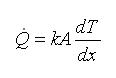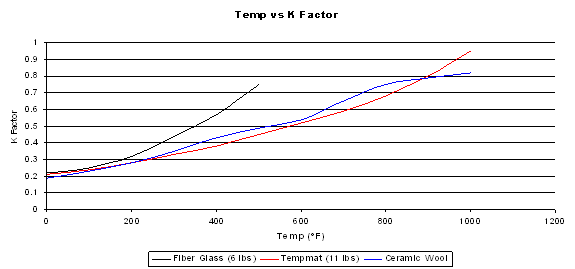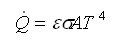## How it Works

Heat is the transport of energy due to a temperature difference between different amounts of matter. Heat can leave the system through conduction, convection, and radiation. With respect to heat transfer, when the amount of heat entering a system and leaving the system is the same the temperature will be at a steady state. Insulation lowers the heat transfer rate by adding a material to the system with a low conductivity (K Factor) and thus increasing steady state temperature and minimizing heat loss.

The transfer of energy that involves molecular collisions is called conduction. Conduction increases with the temperature difference and the ability of the substance to make the transfer (K Factor). Heat transfer due to conductive is expressed by Fourier’s law of conduction. Fourier’s law states that the rate of heat transfer is proportional to the conductivity (k), the surface area (A), and the temperature gradient (dT/dx).The figure below shows the K Factor vs temperature for a few common insulation materials.In flowing mediums, a different heat transfer takes place called convection. Convection includes medium properties, flows, and geometries. Convection accounts for the heat lost to the wind. Heat transfer due to convection is expressed by Newton’s law of cooling. Newton’s law of cooling states that the rate of heat transfer is proportional to the heat transfer coefficient (h), the surface area (A), and the difference in temperature of the two mediums (∆T).The final mode of heat transfer is radiation, which transmits energy as electromagnetic waves. Surface emission is written as a fraction, emissivity ε, of a perfect black body emission as with the surface temperature, T, and the Stefan-Boltzmann constant, σ.The figure below shows an un-insulated steam pipe and an insulated steam pipe. It can be seen, from the figure below, that the heat conduction for the insulated pipe case is lower than that of the un-insulated case. The lower conduction of the insulation increases the pipe temperature and decreases the insulation surface temperature. The higher pipe temperature decreases the heat transfer rate from the steam to the pipe by lowering the temperature gradient, and at the same time, the lower insulation surface temperature decreases the heat transfer rate from the surface to the air by lowering the temperature difference between the ambient air and the insulation. The net effect of this is that the steady state temperature of steam in an insulated pipe is higher than of the un-insulated pipe and the surface is cooler. The presence of the insulation around the pipe minimizes the energy loss to the surrounding environment.